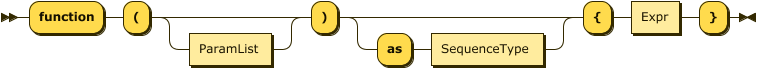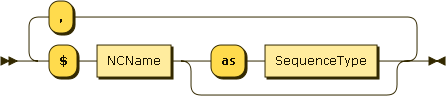#### Inline function expression

JSONiq follows the W3C standard for constructing function items with inline expressions. The following explanations, provided as an informal summary for convenience, are non-normative.

A function can be built directly by specifying its parameters and its body as expression. Types are optional and by default, assumed to be item*.

Function items can also be produced with a partial function application.

Example 39. Inline function expression

```           function (\$x as integer, \$y as integer) as integer { \$x + 2 },
function (\$x) { \$x + 2 }
```

Result. (two function items)

Figure 24. InlineFunctionExprFigure 25. ParamList